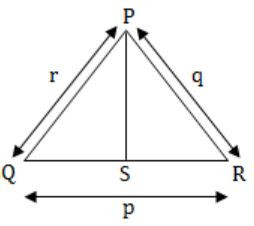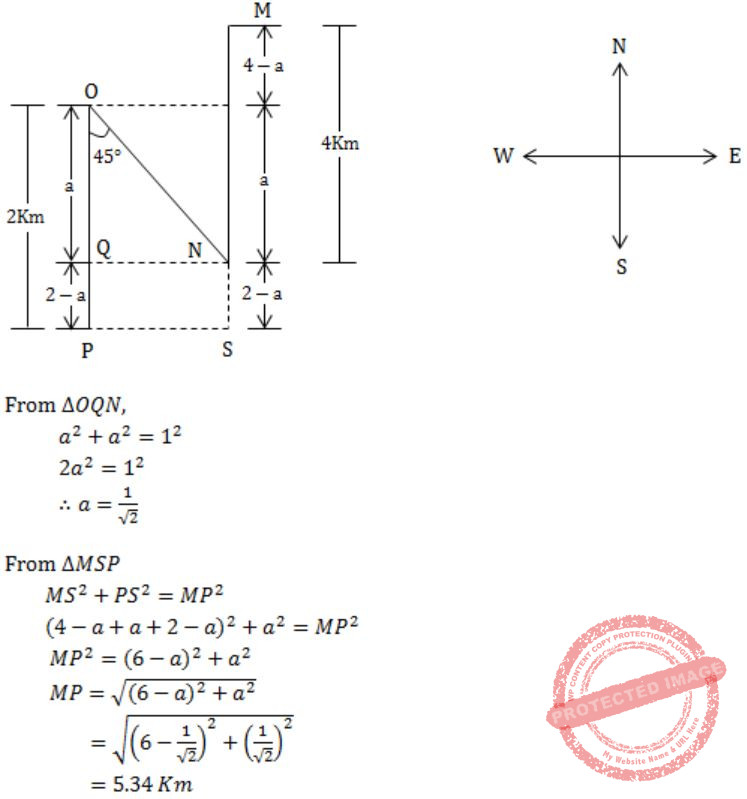## GATE 2015 [Set-2]

 Question 1

Based on the given statements, select the most appropriate option to solve the given question, What will be the total weight of 10 poles each of same weight?

Statements:
(I) One fourth of the weight of a pole is 5 kg
(II) The total weight of these poles is 160 kg more than the total weight of two poles.
 A Statement I alone is not sufficient B Statement II alone is not sufficient C Statement II alone is not sufficient D Both statements I and II together are not sufficient.
Aptitude       Numerical       Video-Explanation
Question 1 Explanation:
Statement-I:
One fourth of the weight of a pole is 5Kg. ⇒ Weight of pole is 4×5 = 20Kg
Weight of 10 poles each of same weight = 10×20 = 200 Kg
∴Statement I alone is sufficient.
Statement-II:
Let, Weight of each pole = W Kg
Given,
10W = 2W + 160
⇒ 8W = 160
W = 20Kg
∴ Weight of each pole = 20 Kg
∴ Weight of 10 poles = 10×20 Kg = 200 Kg
∴ Statement II alone is sufficient.
Either I or II alone is sufficient.
 Question 2

Consider a function f(x) = 1 - |x| on -1 ≤ x ≤ 1. The value of x at which the function attains a maximum and the maximum value of the function are

 A 0, -1 B -1, 0 C 0, 1 D -1, 2
Aptitude       Numerical       Video-Explanation
Question 2 Explanation:
f(x) = 1 - |x| on -1 ≤ x ≤ 1
In the given function, it is given as
-|x| ⇒ To obtain the maximum of this function we have to minimize the value -|x| and the minimum value is 0.
∴ Maximum value of f(x) is at f(0) and i.e., f(x) = 1
Maximum value is 1 at x=0.
 Question 3

A generic term that include various items of clothing such as a skirt, a pair of trousers and a shirt is

 A fabric B textile C fibre D apparel
Aptitude       Verbal       Video-Explanation
Question 3 Explanation:
Apparel- clothing, especially outerwear; garments; attire; raiment
 Question 4

Choose the statement where underlined word is used correctly.

 A The industrialist load a personnel jet. B I write my experience in my personnel diary. C All personnel are being given the day off. D Being religious is a personnel aspect.
Aptitude       Verbal       Video-Explanation
Question 4 Explanation:
personnel- people employed in an organization or engaged in an organized undertaking such as military service.
 Question 5

We __________________ our friend’s birthday and we ______________ how to make it up to him.

 A Completely forgot - - - don’t just know B Forgot completely - - - don’t just know C Completely forgot - - - just don’t know D Forgot completely - - - just don’t know
Aptitude       Verbal       Video-Explanation
Question 5 Explanation:
We completely forgot our friend's birthday and we just don't know how to make it up to him.
 Question 6

In a triangle PQR, PS is the angle bisector of ∠QPR and ∠QPS = 60º. What is the length of PS?A (q+r)/qr B qr/(q+r) C √(q2 + r2) D (q+r)2 /qr
Aptitude       Numerical       Video-Explanation
Question 6 Explanation:
Given,∠QPS = 60º
and PS is angle bisector of ∠QPR
⇒ ∠QPS = ∠SPR = 60º
and ∠QPR = 120º
Let, PS = s
Area of ΔPQR = Area of ΔPQS + Area of ΔSPR
1/2 qr Sin∠QPR = 1/2 rs Sin∠QPS + 1/2 sq∠SPR
1/2 qr Sin 120º = 1/2 rs Sin 60º + 1/2 sq 60º
1/2 qr Sin × √3/2 = 1/2 rs × √3/2 + 1/2 × √3/2
qr = rs + sq
∴ s = qr/q+r
PS = qr/q+r
 Question 7

Out of the following four sentences, select the most suitable sentence with respect to grammar and usage.

 A Since the report lacked needed information, it was of no use to them. B The report was useless to them because there were no needed information in it. C Since the report did not contain the needed information, it was not real useful to them D Since the report lacked needed information, it would not have been useful to them.
Aptitude       Verbal       Video-Explanation
Question 7 Explanation:
(B) there was no needed information
(C) not really useful
(D) would not have been
 Question 8

If the list of letters, P, R, S, T, U is an arithmetic sequence, which of the following are also in arithmetic sequence?

I. 2P, 2R, 2S, 2T, 2U
II. P-3, R-3, S-3, T-3, U-3
III. P2, R2, S2, T2, U2
 A I only B I and II C II and III D I and III
Aptitude       Numerical       Video-Explanation
Question 8 Explanation:
If any set of numbers are in a arithmetic sequence and if a common number if added (or) subtracted from each of these numbers, the new set will also be in arithmetic sequence.
Hence, II is an arithmetic sequence.
If the set of numbers is multiplied by the common number, even then the new set will also be in arithmetic sequence.
Hence, I is an arithmetic sequence.
 Question 9

If p, q, r, s are distinct integers such that:

f(p,q,r,s) = max(p,q,r,s)
g(p,q,r,s) = min(p,q,r,s)
h(p,q,r,s) = remainder of (p×q)(r×s) if (p×q)>(r×s) OR remainder of (r×s)(p×q) if (r×s)>(p×q)

Also a function fgh(p,q,r,s) = f(p,q,r,s) × g(p,q,r,s) ×h(p,q,r,s)

Also the same operations are valid with two variable functions of the form f(p,q).

What is the value of fg(h(2,5,7,3),(4,6,8)?

 A 8 B 9 C 7 D 6
Aptitude       Numerical       Video-Explanation
Question 9 Explanation:
h(2,5,7,3) = remainder of (7×3)/(2×5)
= remainder of 21/10
= 1
fg(1,4,6,8) = f(1,4,6,8) × g(1,4,6,8)
= max(1,4,6,8) × min(1,4,6,8)
= 8 × 1
= 8
 Question 10

Four branches of a company are located at M, N, O and P. M is north of N at a distance of 4km; P is south of O at a distance of 2 km; N is southeast of O by 1 km. What is the distance between M and P in km?

 A 5.34 B 6.74 C 28.5 D 45.49
Aptitude       Numerical       Video-Explanation
Question 10 Explanation:Question 11

An unordered list contains n distinct elements. The number of comparisons to find an element in this list that is neither maximum nor minimum is

 A Θ(nlog n) B Θ(n) C Θ(log n) D Θ(1)
Aptitude       Numerical       Video-Explanation
Question 11 Explanation:
Consider first three element of the list, atleast one of them will be neither minimum nor maximum. ∴ Θ(1)
 Question 12

Let R be the relation on the set of positive integers such that aRb if and only if a and b are distinct and have a common divisor other than 1. Which one of the following statements about R is true?

 A R is symmetric and reflexive but not transitive B R is reflexive but not symmetric and not transitive C R is transitive but not reflexive and not symmetric D R is symmetric but not reflexive and not transitive
Engineering-Mathematics       Set-Theory       Video-Explanation
Question 12 Explanation:
Reflexive:
In aRb, 'a' and 'b' are distinct. So it can never be reflexive.
Symmetric:
In aRb, if 'a' and 'b' have common divisor other than 1, then bRa, i.e., 'b' and 'a' also will have common divisor other than 1. So, yes symmetric.
Transitive:
Take (3, 6) and (6, 2) elements of R. For transitivity (3, 2) must be the element of R, but 3 and 2 don't have a common divisor. So not transitive.
 Question 13

Consider the following transaction involving two bank account x and y.

The constraint that the sum of the accounts x and y should remain constant is that of

 A Atomicity B Consistency C Isolation D Durability
Database-Management-System       Transactions       Video-Explanation
Question 13 Explanation:
The consistency property ensures that the database remains in a consistent state before the (start of the transaction and after the transaction is over. Here sum of the accounts x & y should remain same before & after execution of the given transactions which refers to the consistency of the sum.
 Question 14

A binary tree T has 20 leaves. The number of nodes in T having two children is _________.

 A 19 B 20 C 21 D 22
Data-Structures       Binary-Trees       Video-Explanation
Question 14 Explanation:
Let the number of vertices of a binary tree with "p" leaves be n then the tree has
(i) p vertices (i.e., leaves) of degree 1
(ii) one vertex (i.e., root of T) of degree 2
(iii) 'n - p - 1' (i.e., interval) vertices of degree 3
(iv) n - 1 edges
∴ By Handshaking theorem,
p × 1 + 1 × 2 + (n - p - 1) × 3 = 2(n - 1)
⇒n = 2p - 1
= 39 as p = 20
∴ n - p = 19 vertices have exactly two children
 Question 15

Consider the basic COCOMO model where E is the effort applied in person-months, D is the development time in chronological months, KLOC is the estimated number of delivered lines of code (in thousands) and abbbcbdb have their usual meanings. The basic COCOMO equations are of the form

 A E = a b(KLOC)exp (b b, D = c b(E)exp (d b) B E = a b(KLOC)exp (b b, D = c b(E)exp (d b) C E = a bexp⁡(b b), D = c b(KLOC)exp (d b) D E = a bexp(D b), D = c b(KLOC)exp (b b)
Software-Engineering       COCOMO-Model       Video-Explanation
Question 15 Explanation:
Basic COCOMO model takes the form
Effort applied (E) = ab(KLOC)bb
Development time (D) = cb(E)db
 Question 16

Consider the following statements:

S1: If a candidate is known to be corrupt, then he will not be elected.
S2: If a candidate is kind, he will be elected.

Which one of the following statements follows from S1 and S2 as per sound inference rules of logic?

 A If a person is known to corrupt, he is kind B If a person is not known to be corrupt, he is not kind C If a person is kind, he is not known to be corrupt D If a person is not kind, he is not known to be corrupt
Engineering-Mathematics       Prepositional-Logic       Video-Explanation
Question 16 Explanation:
Let p: candidate known to be corrupt
q: candidate will be elected
r: candidate is kind
then S1 = p→~q
= q→~p (conrapositive rule)
and S2: r→q ⇒ r→~p (transitive rule)
i.e., If a person is kind, he is not known to be corrupt. ∴ Option is C
There are 16 questions to complete.

Register Now